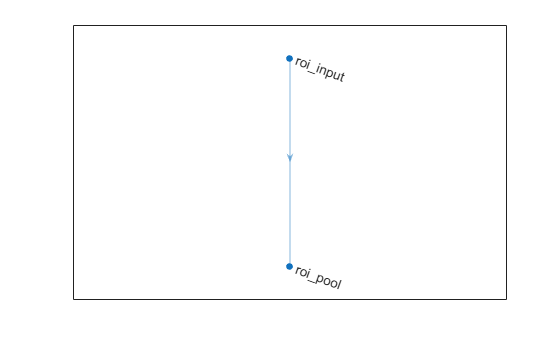Documentation

### This is machine translation

Mouseover text to see original. Click the button below to return to the English version of the page.

Note: This page has been translated by MathWorks. Click here to see
To view all translated materials including this page, select Country from the country navigator on the bottom of this page.

# roiInputLayer

ROI input layer for Fast R-CNN

## Description

An ROI input layer inputs images to a Fast R-CNN object detection network.

## Creation

### Syntax

``layer = roiInputLayer``
``layer = roiInputLayer('Name',Name)``

### Description

``layer = roiInputLayer` creates an ROI input layer.`

example

````layer = roiInputLayer('Name',Name)` creates an ROI input layer and sets the optional `Name` property.```

## Properties

expand all

Layer name, specified as a character vector or a string scalar. To include a layer in a layer graph, you must specify a nonempty unique layer name. If you train a series network with the layer and `Name` is set to `''`, then the software automatically assigns a name to the layer at training time.

Data Types: `char` | `string`

Number of outputs of the layer. This layer has a single output only.

Data Types: `double`

Output names of the layer. This layer has a single output only.

Data Types: `cell`

## Examples

collapse all

Create an ROI input layer.

`roiInput = roiInputLayer('Name','roi_input');`

Create an ROI max pooling layer with output size [4 4].

```outputSize = [4 4]; roiPool = roiMaxPooling2dLayer(outputSize,'Name','roi_pool');```

Add the layers to a LayerGraph.

```lgraph = layerGraph; lgraph = addLayers(lgraph,roiInput); lgraph = addLayers(lgraph,roiPool);```

Specify that the output of the ROI input layer is the `'roi'` input of the ROI max pooling layer.

```lgraph = connectLayers(lgraph,'roi_input','roi_pool/roi'); plot(lgraph)```Download ebook